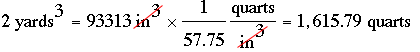SEARCH HOMEMath Central Quandaries & QueriesQuestion from Mary: I need 2 yards of soil, how many quarts is this?Hi Mary,

I looked up quart on Wikipedia and found that one US quart is 57.75 cubic inches. A yard is 36 inches so a cubic yard is 36 × 36 × 36 = 46656 cubic inches. Thus 2 cubic yards is 93312 cubic inches. ThusYou can also ask Google to do this for you. Go to Google and type 2 cubic yards in quarts into the search window and Google will respond with 2 (cubic yards) = 1 615.79221 US quarts.

PennyMath Central is supported by the University of Regina and The Pacific Institute for the Mathematical Sciences.